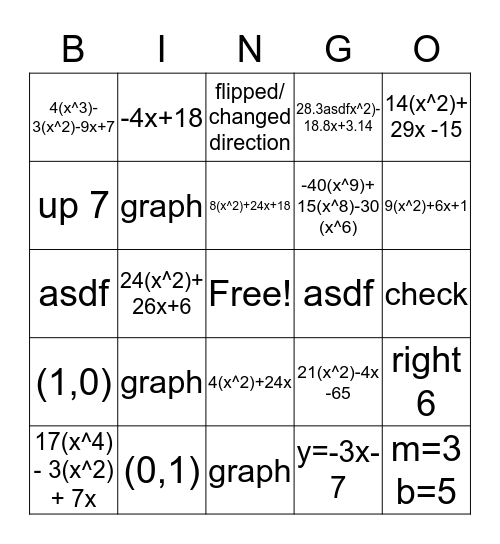# EXAM REVIEWThis bingo card has a free space and 26 words: 4(x^2)+24x, y=-3x-7, 8(x^2)+24x+18, (0,1), 14(x^2)+ 29x -15, 4(x^3)- 3(x^2)-9x+7, up 7, -4x+18, check, 21(x^2)-4x -65, -40(x^9)+ 15(x^8)-30 (x^6), asdf, right 6, 17(x^4) - 3(x^2) + 7x, 24(x^2)+ 26x+6, (0,-5), 28.3asdfx^2)- 18.8x+3.14, (1,0), flipped/ changed direction, m=3 b=5, 9(x^2)+6x+1, graph, asdf, graph, graph and graph.

⚠ This card has duplicate items: asdf (2), graph (4)

## Play Online